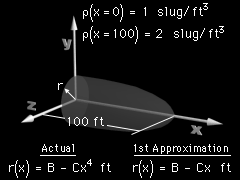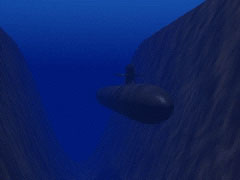Ch 7. Centroid/Distributed Loads/Inertia Multimedia Engineering Statics Centroid: Line Area Vol Centroid: Composite Distributed Loads Area Moment of Inertia
 Chapter 1. Basics 2. Vectors 3. Forces 4. Moments 5. Rigid Bodies 6. Structures 7. Centroids/Inertia 8. Internal Loads 9. Friction 10. Work & Energy Appendix Basic Math Units Sections Search eBooks Dynamics Fluids Math Mechanics Statics Thermodynamics Author(s): Kurt Gramoll ©Kurt GramollSTATICS - CASE STUDY IntroductionGeometry Diagram In order to determine a submarine's buoyancy characteristics, designers must accurately determine the centroid, center of mass, and center of gravity for various parts of the sub. What is known: The radius of the nose cone is given by r(x) = B - CxD. The length of the nose cone is 100 ft. The base of the nose cone has a radius 20 ft. The shape exponent D is 4, but as a first approximation, the designers use D = 1 in order to simplify the mathematics. The density of the nose cone varies linearly from 1 slug/ft3 at the base to 2 slug/ft3 at the tip. QuestionSubmarine Path Graphic As a first approximation, assume the nose code is a simple cone shape (D = 1 in the above equation). Where is the centroid, center of mass, and center of gravity? Approach Use symmetry to determine the y and z locations of the centroid, center of mass, and center of gravity. Solve for the x location by integrating the appropriate quantities from the base to the tip of the nose cone.

Practice Homework and Test problems now available in the 'Eng Statics' mobile app
Includes over 500 problems with complete detailed solutions.
Available now at the Google Play Store and Apple App Store.